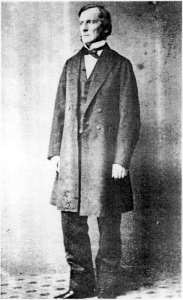# George Boole

The English mathematician and philosopher George Boole (1815-1864) was one of the first men, after the great Gottfried Leibniz, who believed that the human thinking is mastered by laws, which can be described by means of mathematic. Boole is the inventor of Boolean logic, which is the basis of modern digital computer logic, thus Boole is regarded in hindsight as a founder of the field of computer science.

The child prodigy and self-taught genius George Boole first became interested in mathematics as a tool to solve mechanical problems in his instrument making occasions. His interest quickly blossomed and he soon began an elaborate project of self-education in mathematics. In 1838 Boole wrote his first mathematical paper. In 1841 he founded a new branch of mathematics called Invariant Theory, later to inspire Einstein. Boole was awarded the first Gold Medal of the Royal Society of London in 1844 for a paper on Differential Equations, whose methods are still used today.Speculations concerning a calculus of reasoning and applying the algebra to the solution of logical problems had at different times occupied thoughts of the great mathematician, but it was not till the spring of 1847, that he put his ideas into the essay, called Mathematical Analysis of Logic. It was the ground-breaking work that laid the foundations for what is known today as Boolean algebra and the propositional calculus. It not only expanded on Gottfried Leibniz's earlier speculations on the correlation between logic and math, but argued that logic was principally a discipline of mathematics, rather than philosophy.

It seems Boole have been motivated in his research by his intense religious convictions. At the age of 17 he had a mystic experience in which he felt God called on him to explain how the mind processes thought. He decided to do this in a mathematical form, for the Glory of God.

Boole afterwards regarded the Mathematical Analysis of Logic as a hasty and imperfect exposition of his logical system, and he desired that his much larger work. In 1854 he wrote the An Investigation of the Laws of Thought, on which are founded the Mathematical Theories of Logic and Probabilities, should alone be considered as containing a mature statement of his views.

Boole did not regard logic as a branch of mathematics, as the title of his earlier essay might be taken to imply, but he pointed out such a deep analogy between the symbols of algebra and those that can be made, in his opinion, to represent logical forms and syllogisms, that we can hardly help saying that (especially his) formal logic is mathematics restricted to the two quantities, 0 and 1.

Boole proposed that logical propositions should be expressed as algebraic equations. The algebraic manipulation of the symbols in the equations provides a fail-safe method of logical deduction, i.e. logic can be reduced to algebra. He replaced the operation of multiplication by the word AND and addition by the word OR. The symbols in the equations can stand for collections of objects (sets) or statements in logic. For example, if x is the set of all pink pigs and y is the set of all fat pigs, then x+y is the set of all pigs that are pink or fat, and xy is the set of all pigs that are pink and fat.

Similarly, if z = the set of all Hampshire pigs, then z(x+y) = zx+zy, in other words the set of Hampshire pigs that are either pink or fat is the same as the collection of pigs, that are Hampshire and pink or Hampshire and fat.

Why is the Boolean algebra so important for the computer science and digital circuitry?

Boolean algebra provides the basis for analyzing the validity of logical propositions because it captures the two-valued character (binary) of statements that may be either true or false.

In 1930s, a number of researchers noticed that Boole's two valued logic lent itself to a description of electrical switching circuits. They showed that the binary numbers (0 and 1), combined through Boolean algebra, could be used to analyze electrical switching circuits and thus used to design electronic computers. Today, digital computers and electronic circuits are designed to implement this binary arithmetic.